基于ANSYS Workbench半导体激光器封装热特性分析Analysis of Thermal Characteristics of Semiconductor Laser Packaging Based on ANSYS Workbench

• 全文下载: PDF(760KB)    PP.232-238   DOI: 10.12677/MP.2018.84025
• 下载量: 337  浏览量: 1,016   科研立项经费支持

Based on the theoretical analysis of the thermal characteristics of semiconductor lasers, the model of c-mount packaging is established by using ANSYS Workbench software. The temperature and heat flux distribution of single-tube semiconductor laser chips are simulated and analyzed. When the output power is 10 w, the temperature of traditional c-mount packaging chips is 66.393˚C, and thermal resistance is 4.6 k/w. By adding a layer of graphene with high thermal conductivity be-tween the heat sink and the laser chip, the heat flux diffusion area is increased; the temperature of the chip is reduced to 55.587˚C; the thermal resistance is 3.5 k/w; and the heat dissipation effect is obvious. The maximum output power is increased from 15.4 w to 18.5 w. It is found that the output power is increased by 20%.

1. 引言

2. 理论分析

1) 当电流注入有源区且小于阈值电流时，器件发出微弱的自发辐射光，没有激光产生，这部分注入的电流主要产生废热，热功率密度为QNR

${Q}_{NR}=\frac{1}{d}Uj\left(1-{\eta }_{i}\right)$ (1)

${Q}_{act}=\frac{{V}_{j}\left(1-{\eta }_{sp}{f}_{sp}\right)}{{d}_{act}}\left[{j}_{th}+\left(j-{j}_{th}\right)\left(1-{\eta }_{i}\right)\right]$ (2)

${T}_{j}={T}_{sink}+\left(IV-P\right){R}_{th}$ (3)

${I}_{th}={I}_{th}\left({T}_{r}\right)\mathrm{exp}\left(\frac{T-{T}_{r}}{{T}_{s}}\right)$ (4)

2) 除有源区外各层外延材料的损耗及电极层的电阻生成的热。(5)

Q为热功率密度，pi为每层材料的电阻率。

3) 半导体激光激光器稳定的工作后的热传导方程为：

$K\left(\frac{{\partial }^{2}T}{\partial {x}^{2}}+\frac{{\partial }^{2}T}{\partial {y}^{2}}+\frac{{\partial }^{2}T}{\partial {z}^{2}}\right)+Q=0$ (6)

$\eta =\frac{P-{P}_{th}}{I-{I}_{th}{h}_{v}}$ (7)

$P={P}_{th}+\frac{{\eta }_{ex}{E}_{p}}{e}\left(I-{I}_{th}\right)$ (8)

Pth为阈值电流下激光器光功率，ηex外微量子效率，Ep为光子能量，e为电子电荷，I为激光器驱动电流，Ith为阈值电流。

$\eta =\frac{P}{IV+{I}^{2}r}$ (9)

1) 半导体激光器工作过程中，内部的电子-空穴非辐射复合转换成光子产生的热量为主要热源。

2) 半导体激光器芯片腔长1.8 mm，热功率为10 W，热功率密度为7.407 × 109 W/m3，且各个外延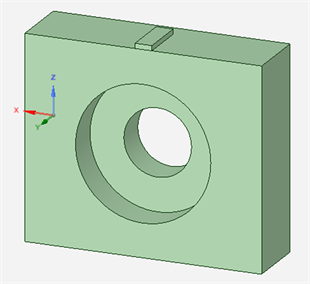Figure 1. Workbench Model of c-mount package structureTable 1. Material parameters of the laser

3) 由于激光器芯片尺寸很小且非常薄，模拟过程计算温度不高，忽略芯片各个面的热辐射及空气对流散热。

4) 模拟计算中热沉下表面恒温20℃，同时选择两种热导率不同的焊料AuSn焊料和SnAgCu焊料对比焊料厚度及焊料热导率不同对半导体激光器的影响。

3. 模拟结果分析

${R}_{th}=\frac{T-{T}_{0}}{P}$ (10)Figure 2. Relationship between solder thickness and temperature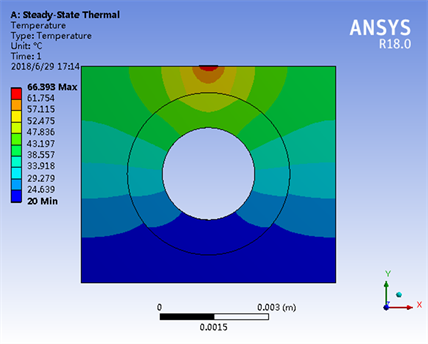Figure 3. Laser temperature distribution cloud imageTable 2. Graphene layer parameter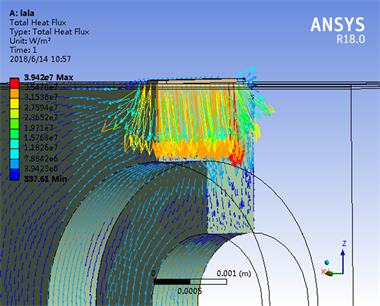Figure 4. Heat flux vector diagram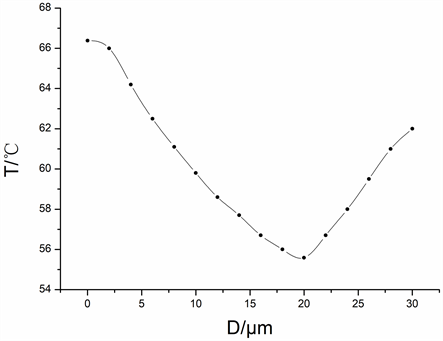Figure 5. Relationship between graphene layer thickness and chip temperature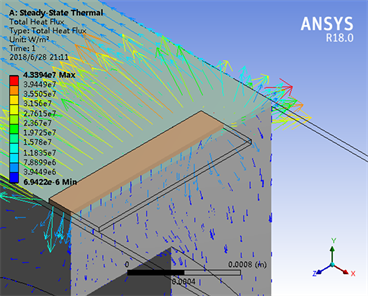Figure 6. Heat flux vector diagram of the improved structureFigure 7. P-I Characteristics with different thermal resistance

4. 结论

NOTES

*通讯作者。

  Lu, Y., Nie, Z., Zhang, P., et al. (2016) Numerical Simulation of Thermo-Mechanical Behavior in High Power Diode Laser Arrays. 17th International Conference on Electronic Packaging Technology, Wuhan, 16-19 August 2016, 76-83.  王狮凌, 房丰洲. 大功率激光器及其发展[J]. 激光与光电子学进展, 2017, 54(9): 45-58.  Pascoe, N. (2011) Reliability Technology: Principles and Practice of Failure Prevention in Electronic Systems. Studies in Phi-losophy & Education, 9, 313-329. https://doi.org/10.1002/9780470980101  范嗣强. 大功率半导体激光器热沉技术的研究现状[J]. 激光杂志, 2018(2): 14-19.  王文, 许留洋, 王云华, 等. 热沉尺寸对半导体激光器有源区温度的影响[J]. 半导体光电, 2013, 34(5).  杨宏宇, 刘林, 舒世立, 等. 大功率半导体激光器有源区温度影响因素分析[J]. 江苏科技大学学报(自然科学版), 2017, 31(2): 143-147.  张晓磊, 薄报学, 张哲铭, 等. C-mount封装激光器热特性分析与热沉结构优化研究[J]. 发光学报, 2017, 38(7): 891-896.  陆日, 许留洋, 高欣, 等. 电注入椭圆微腔半导体激光器热特性分析[J]. 中国激光, 2016(4): 42-47.  全伟, 李光慧, 陈熙, 等. 一体化半导体激光器的ANSYS热仿真及结构设计[J]. 光学精密工程, 2016, 24(5): 1080-1086.  Fan, W. and Liu, X. (2017) Advancement in High Thermal Conductive Graphite for Microelectronic Packaging. RF and Microwave Microelectronics Packaging II. Springer International Publishing.